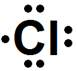# Electron Dot Structures Formula

Electron Dot Structures Formula

Electron dot structures show the number of valence electrons for that element as dots. The valence electrons are those that occupy the highest energy level which can be obtained using the periodic table. For example, the elements in group IA of the periodic table have 1 valence electron.

An electron dot structure is depicted using the element symbol and then starting at the top of the element symbol, dots are added in a clockwise fashion until the number of valence electrons has been reached. The maximum number of electrons is limited to eight.

Electron Dot Structures Formula Questions:

1. Give the electron dot structure for aluminum.

Answer:

Aluminum is in group IIIA of the periodic table therefore it has three valence electrons. The symbol for aluminum is Al which will be surrounded by three dots.2. Give the electron dot structure of chlorine.

Answer:

Chlorine is in group VIIA of the periodic table therefore it has seven valence electrons. The symbol for chlorine is Cl which will be surrounded by seven dots.Related Links: